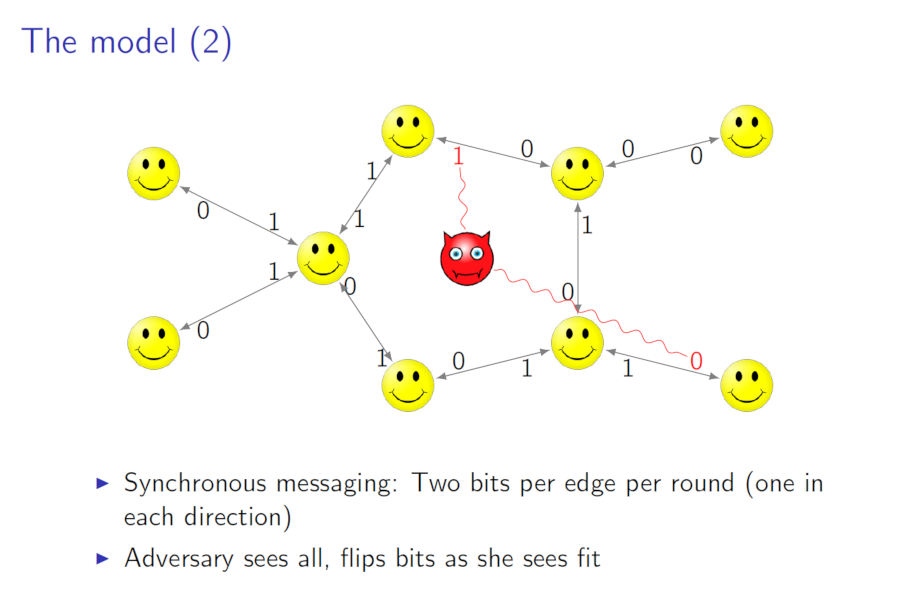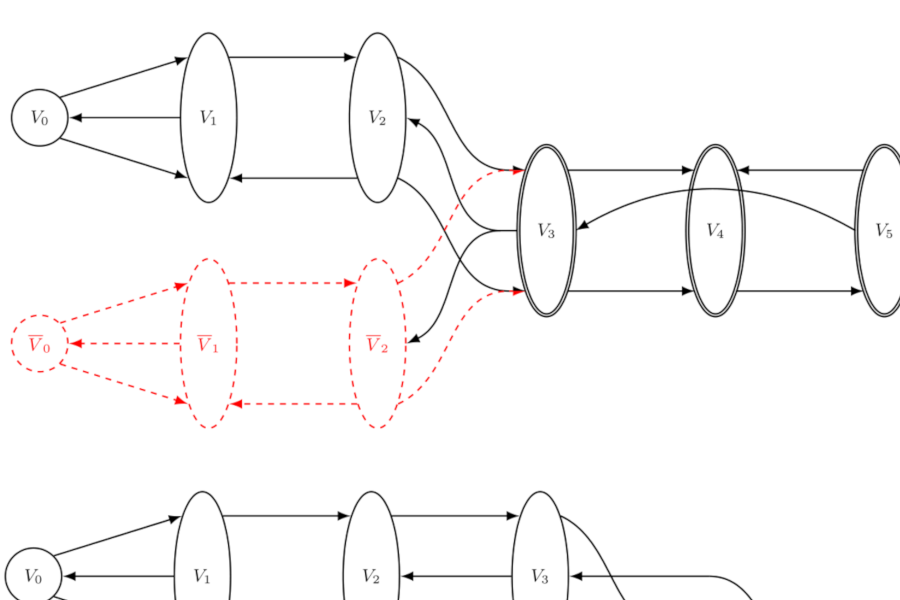Back to list of papers

# The Adversarial Noise Threshold for Distributed Protocols

By William M. Hoza and Leonard Schulman

Abstract (for specialists)

We consider the problem of implementing distributed protocols, despite adversarial channel errors, on synchronous-messaging networks with arbitrary topology.

In our first result we show that any $$n$$-party $$T$$-round protocol on an undirected communication network $$G$$ can be compiled into a robust simulation protocol on a sparse ($$\mathcal{O}(n)$$ edges) subnetwork so that the simulation tolerates an adversarial error rate of $$\Omega(\frac{1}{n})$$; the simulation has a round complexity of $$\mathcal{O}\left(\frac{m \log n}{n} T\right)$$, where $$m$$ is the number of edges in $$G$$. (So the simulation is work-preserving up to a log factor.) The adversary's error rate is within a constant factor of optimal. Given the error rate, the round complexity blowup is within a factor of $$\mathcal{O}(k \log n)$$ of optimal, where $$k$$ is the edge connectivity of $$G$$. We also determine that the maximum tolerable error rate on directed communication networks is $$\Theta(1/s)$$ where $$s$$ is the number of edges in a minimum equivalent digraph.

Next we investigate adversarial per-edge error rates, where the adversary is given an error budget on each edge of the network. We determine the limit for tolerable per-edge error rates on an arbitrary directed graph to within a factor of 2. However, the construction that approaches this limit has exponential round complexity, so we give another compiler, which transforms $$T$$-round protocols into $$\mathcal{O}(mT)$$-round simulations, and prove that for polynomial-query black box compilers, the per-edge error rate tolerated by this last compiler is within a constant factor of optimal.

Not-so-abstract (for curious outsiders)

⚠️ This summary might gloss over some important details.

Imagine that many people (or computers) are connected by a network of communication channels. They would like to have a conversation, but unfortunately, an adversary is altering their messages to confuse them. The parties may deal with this noise by encoding their conversation in whatever way they like. In this paper, we determine the maximum amount of noise that can be tolerated in a couple different models as a function of the particular communication network.

We posted a manuscript online in December 2014; I presented the paper at SODA in January 2016. I recommend that you read the SODA proceedings version. The SODA reviewers' feedback never got incorporated into the arXiv version.

Expository material:Slides from my presentation at SODA (January 2016).Poster from my presentation at Caltech's CMS "Celebration of Undergraduate Research" (May 2015).

🔭

Ran Gelles wrote a survey that includes a summary of this paper (Section 7.3).